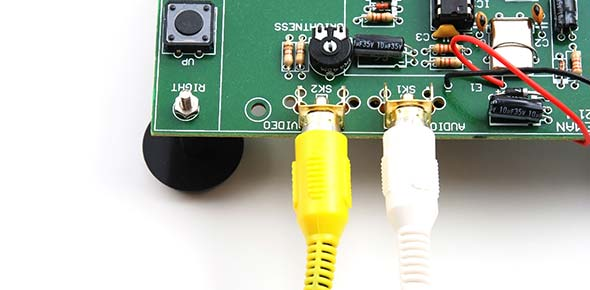# Es&t017

10 Questions | Total Attempts: 293SettingsThis QUIZ is made or by product of my continuous research & study about Electronics Engineering to help the Electronics Engineer/Technician Aspirants in passing the Board Examinations.

• 1.
The real part of an antenna's input impedance is due to:
• A.

• B.

The reflected signal

• C.

The SWR

• D.

All of the above

• 2.
A half-wave dipole is sometimes called:
• A.

A Marconi antenna

• B.

A Hertz antenna

• C.

A Yagi antenna

• D.

None of the above

• 3.
The end-to-end length of a half-wave dipole antenna is actually:
• A.

One wavelength

• B.

One half-wavelength

• C.

Slightly longer than a half-wavelength

• D.

Slightly shorter than a half-wavelength

• 4.
The radiation of energy from an antenna can be seen in the:
• A.

Standing wave pattern around the antenna

• B.

SWR along the feed cable

• C.

• D.

Power loss of the antenna

• 5.
Measured on the ground, the field strength of a horizontally polarized half-wave dipole antenna is strongest:
• A.

In one direction

• B.

In two directions

• C.

In all directions

• D.

Depends on the number of elements

• 6.
The ability of an antenna to radiate more energy in one direction than in other directions is called:
• A.

Directivity

• B.

Selectivity

• C.

Active antenna

• D.

Resonance

• 7.
The front-to-back ratio of a half-wave dipole antenna is:
• A.

0 dB

• B.

3 dB

• C.

10 dB

• D.

Infinite

• 8.
An antenna's beamwidth is measured:
• A.

From +90° to –90°

• B.

From front to back

• C.

Between half-power points

• D.

Between the minor side-lobes

• 9.
ERP stands for:
• A.

• B.

• C.

• D.

• 10.
"Ground Effects" refers to the effects on an antenna's radiation pattern caused by:
• A.

Radio signals reflecting off the ground

• B.

Buildings and other structures on the ground

• C.Back to top